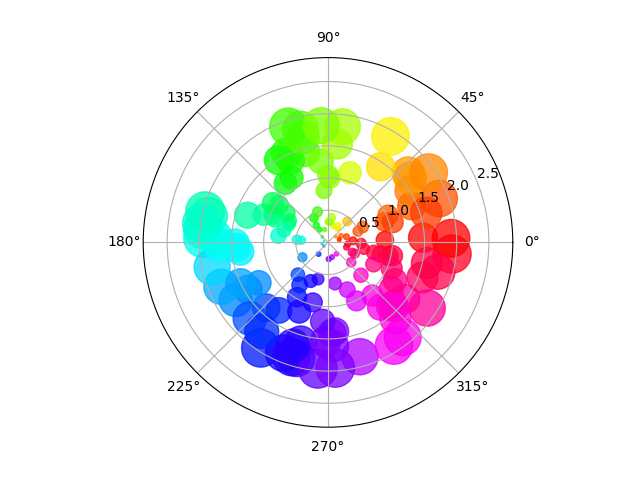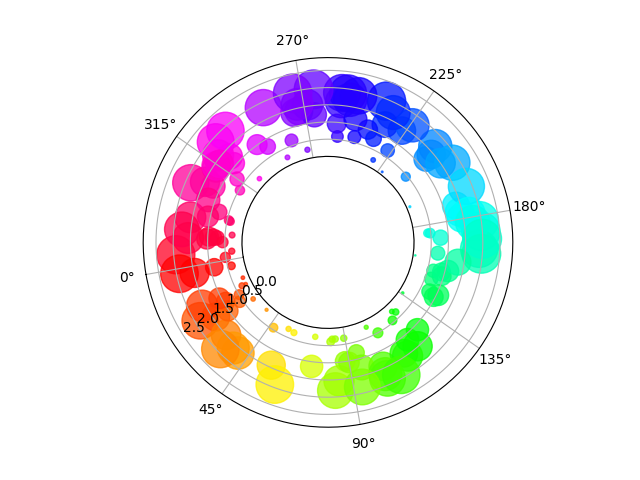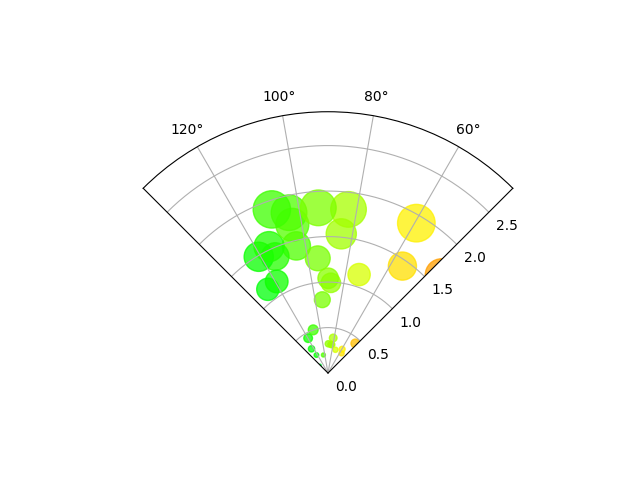You are reading an old version of the documentation (v3.0.0). For the latest version see https://matplotlib.org/stable/gallery/pie_and_polar_charts/polar_scatter.html

# Scatter plot on polar axis¶

Size increases radially in this example and color increases with angle (just to verify the symbols are being scattered correctly).

```import numpy as np
import matplotlib.pyplot as plt

# Fixing random state for reproducibility
np.random.seed(19680801)

# Compute areas and colors
N = 150
r = 2 * np.random.rand(N)
theta = 2 * np.pi * np.random.rand(N)
area = 200 * r**2
colors = theta

fig = plt.figure()
ax = fig.add_subplot(111, projection='polar')
c = ax.scatter(theta, r, c=colors, s=area, cmap='hsv', alpha=0.75)
```## Scatter plot on polar axis, with offset origin¶

The main difference with the previous plot is the configuration of the origin radius, producing an annulus. Additionally, the theta zero location is set to rotate the plot.

```fig = plt.figure()
ax = fig.add_subplot(111, polar=True)
c = ax.scatter(theta, r, c=colors, s=area, cmap='hsv', alpha=0.75)

ax.set_rorigin(-2.5)
ax.set_theta_zero_location('W', offset=10)
```## Scatter plot on polar axis confined to a sector¶

The main difference with the previous plots is the configuration of the theta start and end limits, producing a sector instead of a full circle.

```fig = plt.figure()
ax = fig.add_subplot(111, polar=True)
c = ax.scatter(theta, r, c=colors, s=area, cmap='hsv', alpha=0.75)

ax.set_thetamin(45)
ax.set_thetamax(135)

plt.show()
```